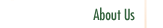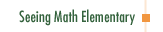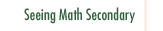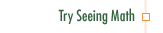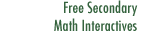Number & Operations: Broken Calculator

In Number & Operations: Broken Calculator, students develop computational fluency by solving problems with a "broken" calculator. For example, a baker packs 315 buns into boxes of 8. How many boxes will the baker need? The division key is broken! Students must find ways to solve the problem, evaluate the solutions, and explain their reasoning.

Participants in this course explore:

• Ways to challenge students to create strategies for solving problems and to evaluate mathematical ideas.
• The importance of having students explain mathematical reasoning to each other.
• Alternative strategies for solving multi-digit multiplication and division problems, from repeated addition to decomposition and compensation strategies.
• Problem-solving activities that help students develop strong computational skills and number sense.
• The calculator as a problem-posing tool.
• Questioning strategies that foster resourceful problem solving.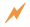Click to Get Seeing Math™ ElementaryCourse Listing Effective Questioning in the Mathematics Classroom Foundations of EffectiveMathematics Teaching Formative Assessment in the Mathematics Classroom Number & Operations: Division with Remainders Number & Operations: The Magnitude of Fractions Number & Operations: Broken Calculator Geometry: 2D and 3D Figures Geometry: Calculating Area of a Triangle Data Analysis and Probability: Using Data to Make Predictions Data Analysis and Probability: Measures of Center Pre-Algebra: Pan Balance Equations Pre-Algebra: Patterns and Functions